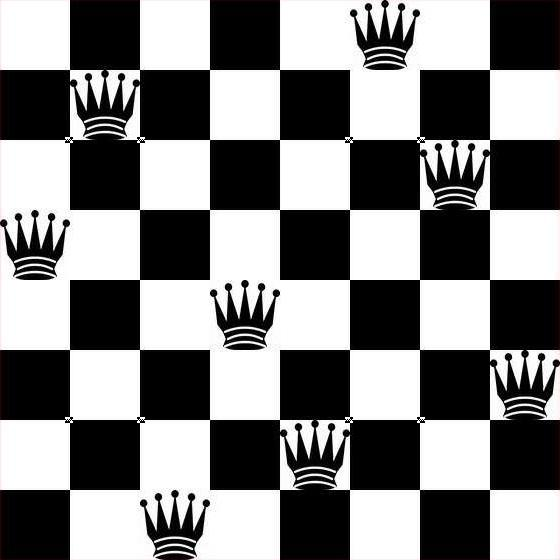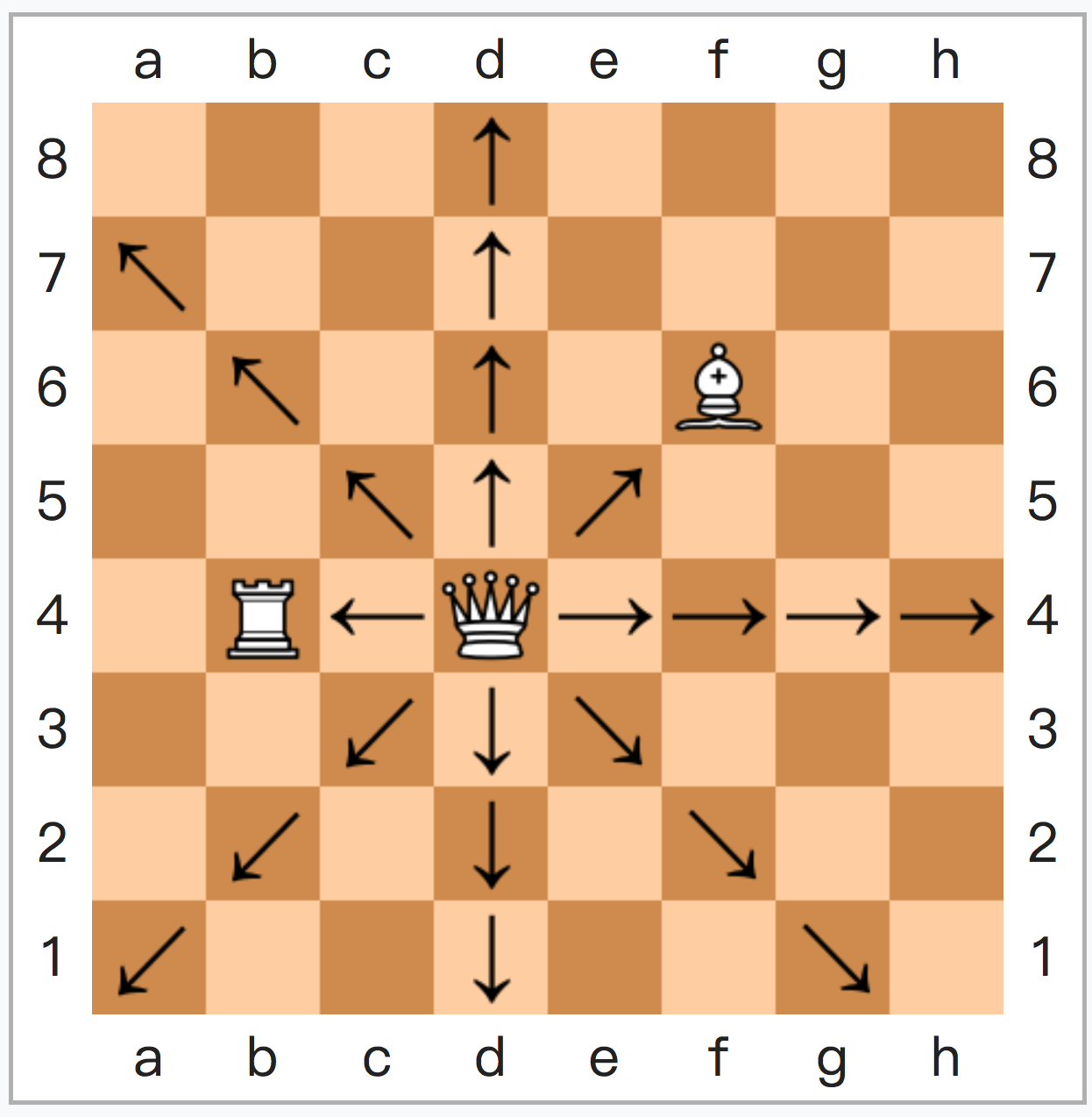# N个女人的老死不相往来——n皇后问题n皇后问题，较早为八皇后问题，出现于1848年。
Max Bezzel讨论的是“在国际象棋上出现8个后时，（八皇后问题的其中一个解）

（一方全升变，一方被吃过路兵一次，加上初始两个）

——直到图论和函数式编程思想出现，

' n queens cast by go basic
[loop]
input "How many queens (N>=4)";n
if n < 4 then
print "Must be greater than 4"
goto [loop]
end if

dim plot$(100,100) dim q(n+20) dim e(n+20) dim o(n+20) r=n mod 6 if r<>2 and r<>3 then gosub [samp] goto [shoBoard] end if for i=1 to int(n/2) e(i) = 2 * i next for i=1 to int((n/2)+.5) o(i) = 2 *i-1 next if r = 2 then gosub [edt2] if r = 3 then gosub [edt3] s = 1 for i=1 to n if e(i)>0 then q(s) = e(i) s = s+1 end if next for i=1 to n if o(i) > 0 then q(s) = o(i) s = s + 1 end if next ' print board [shoBoard] cls for i = 1 to n plot$(i,26-q(i)) = "*"
plot$(i,24-n) = chr$(96+i)
plot$(n+1,26-i) = str$(i)
next i
for ii = 1 to 100
for jj = 1 to 100
print left$(plot$(jj,ii)+" ",1);
next jj
print
next ii
end

' the simple case
[samp]
p = 1
for i = 1 to n
if i mod 2=0 then
q(p) = i
p    = p + 1
end if
next i
for i = 1 to n
if i mod 2 then
q(p) = i
p    = p + 1
end if
next
return
' edit list when remainder is 2
[edt2]
for i=1 to n
if o(i) = 3 then
o(i) = 1
else
if o(i)=1 then o(i) = 3
end if
if o(i) = 5 then
o(i)= o(i) -1
else
if o(i) = 0 then
o(i) = 5
return
end if
end if
next

' edit list when remainder is 3
[edt3]
for i = 1 to n
if e(i) = 2 then
e(i)  = e(i)-1
else
if e(i) = 0 then
e(i) = 2
goto [more]
end if
end if
next i
' edit list some more
[more]
for i = 1 to n
if (o(i)=1 or o(i)=3) then
o(i) = o(i)-1
else
if o(i) = 0 then
o(i)   = 1
o(i+1) = 3
return
end if
end if
next


# Brute force permutations for 8 queens by R

safe <- function(p) {
n <- length(p)
for (i in seq(1, n - 1)) {
for (j in seq(i + 1, n)) {
if (abs(p[j] - p[i]) == abs(j - i)) return(F)
}
}
return(T)
}

queens <- function(n) {
p <- 1:n
k <- 0
while (!is.null(p)) {
if(safe(p)) {
cat(p, "\n")
k <- k + 1
}
p <- next.perm(p)
}
return(k)
}

queens(8)


——只会利用工具的人，终究会被工具抛弃。

N皇后问题中，穷举法的复杂度为(n!)，1 N皇后于NxN棋盘上，若老死不相往来，则绝不在同一行列。
2 攻击范围有重叠时，绝非边与边重叠，只可相交，且相交仅会出现一次。

（计算第5横行时，下子状态保留为 0001 0000，

#include <iostream>

// total:记录解；finishline:确认的行
int total = 0, finishline = 1;

// row:竖直攻击; ls:斜杠攻击; rs:反斜杠攻击
void trying(long row, long ls, long rs)
{
if (row != finishline) // 开始新行
{
long position = finishline & ~(row | ls | rs);
while(position) // 若行间无位可放...
{
long pointline = position & -position;
position -= pointline;
trying(row + pointline, (ls + pointline) << 1, (rs + pointline) >> 1);
}
}
else
{
total++;
}
}

using namespace std;

int main(int argc, char *argv[]) {
int grid = 8; // 修改grid的话，1～31皇后都能解w

finishline = (finishline << grid) - 1;

trying(0, 0, 0);

cout << total << endl;

return 0;

}


#include <iostream>
int total = 0, finishline = 1;
void trying(long row, long ls, long rs){
if (row != finishline) { long position = finishline & ~(row | ls | rs);
while(position) { long pointline = position & -position; position -= pointline; trying(row + pointline, (ls + pointline) << 1, (rs + pointline) >> 1); }} else {total++;}}
using namespace std;
int main(int argc, char *argv[]) {
int grid = 8; // 修改grid的话，1～31皇后都能解w
finishline = (finishline << grid) - 1; trying(0, 0, 0); cout << total << endl;
return 0;}


// 此处需使用八皇后算法，但忽略计算过程，仅使用答案节省时间。
// 八皇后解枚举数据头在******
void 8queensAnswers (0, 0, 0, 0)
//在里面做一个调用92个答案的接口，传参出去给大结构体
//保存各个答案类似于[91, 0, 4, 7, 5, 2, 6, 1, 3]这样
//或者n皇后的话[0,1][1,0][2,0][3,2][4,10][5,40]...这样


N皇后问题——记录下那些老死不相往来的N个女人的位置吧

1.シズキ says: